# Warehouses

To four warehouses is going cement in 25 kg bags. To first one third, to second quarter of the total. The third store got two thirds of the rest, and the last 310 tons came to fourth. How many cement is in all warehouses and how much got every one?

t (total) =  2232 ton
m1 =  744 ton
m2 =  558 ton
m3 =  620 ton
m4 =  310 ton

### Step-by-step explanation: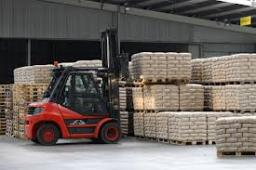Did you find an error or inaccuracy? Feel free to write us. Thank you!Tips to related online calculators
Need help to calculate sum, simplify or multiply fractions? Try our fraction calculator.
Do you have a linear equation or system of equations and looking for its solution? Or do you have a quadratic equation?

## Related math problems and questions:

• Tons of coal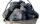Coal hopper has a capacity of 285 liters. How many tons is it? The bulk density of coal is 916 kg/m3.
• Farmers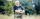Farmers loaded into a truck of fruit and vegetables intended for the store. 10 boxes of 5 kg pears, 8 boxes of 6 kg plums, 7 boxes 9 kg of carrots and 10 bags of 50 kg of potatoes. How many kilograms of fruit and vegetables loaded in total? How many kg co
• Sales storesThe first sales store passed the 1/3 and the second 2/5 of the total amount of goods.In third store passed the 2/3 rest of the goods. The remaining 40 kg of goods put into fourth store. How many kilograms of goods pass to a third store?
• Potatoes bags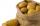I have three bags with 21 kg potatoes. The first bag is 5.5 kg more than in the second bag and the third is 0.5 kg more than in the second bag. Determine how many kgs of potatoes is in each bag.
• Wood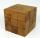Wood cube with edge 11 cm weights 0.753 kg. What weight have 10, 100 and 1000 these cubes?
• Dairy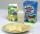Diary workers calculated according to the standards from the 108 litres of milk is possible to produce 9 kg cheese. How many tons of cheese was possible according to standards make from milk from 100 cows devoted for 30 days with average daily milk yield
• A shopkeeper 3A shopkeeper sells 8 1/3 kg, 10 1/4 kg and 11 1/5 kg of apples on 3 consecutive days. What is the total weight of apples sold?
• Locomotive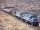The whole loaded train weighs 760 tons. It has twenty wagons, each carrying 20 tons of grain and empty weighs 10 tons. How much weight a locomotive?
• Can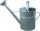Watering can full of water weighs 10 kg. Half-full can weighs 5.5 kg. How much weigh can?
• The bakery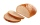The bakery delivered 60 kg of bread to the store, the breads weighed 1 kg and 2 kg, a total of 35 pieces. How many loaves weighed 1 kg and how many were two-kilo loaves?
• Bag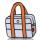Nelly found interesting point. An empty bag weighs 4 kg less than full. And empty bag is 5 times easier than full. How many kg weigh things in this bag?
• Peter 2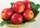Peter wanted to have 120 kilogram of fruits.  He picked 10 bags .  each bags contains 9 1/2 kilograms. How many kilograms he need more?
• Freight train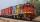Twenty-five identical freight wagons are connected behind the locomotive. The weight of the locomotive and all wagons is 1009000 kg. The locomotive weighs 84 tons. How many kilograms does one freight car weigh?
• One kilogramThe apple weighs 125 grams and half apple. How many apples weigh 1 kilogram?
• Cooks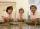Four cooks cleaned 5 kg of potatoes for 10 minutes. How many cook would have to work clean 9 kg of potatoes for 12 minutes?
• Crown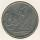Siblings collect a 2-crowns and 5-crowns. Together have 80 coins with a total of value 310 crowns. How many saved two-crowns and how many five-crowns?
• Apples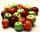Mr. Vesely give to the market his own 48 kg of apples, 40 kg from Mr. Kovar and 72 kg of Mr. Novak. Grossed a total of 1,920 CZK. How must divide income money for apples?